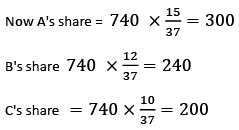Courses

# A, B and C can finish a work in 8 days, 10 days and 12 days respectively. They worked together and got Rs. 740. How much will each of them get ?a)Rs. 200, Rs. 240, Rs. 200b)Rs. 300, Rs. 240, Rs. 200c)Rs. 100, Rs. 200, Rs. 300d)Rs. 340, Rs. 100, Rs. 200Correct answer is option 'B'. Can you explain this answer? Related Test: DU LLB Mock Test- 3

## CLAT QuestionNotes Wala Aug 09, 2021
1 day work of A,B & C is 1/8, 1/10 & 1/12 respectively
Therefore, Their names in money = 1/8 : 1/10 : 1/12 = 15: 12: 10
Sum of their share = 15 + 12 + 10 = 37